# Integral of e^(ax)*sin(x)

In this post we are going to integrate this seemingly hard integral, which in my opinion is actually very interesting!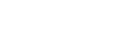When we have a product of a trigonometric and a non trigonometric function, the best technique to try out is integration by parts, which we are going to perform here. What we need to choose are u and dv, but in this case it doesn’t really matter.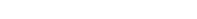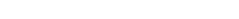As you may know,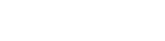Therefore,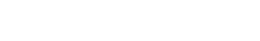This is pretty much the same integral we want to solve, except there is cos(x) instead of sin(x); this means the only thing we can do is perform integration by parts again: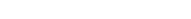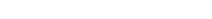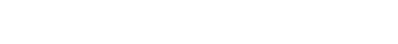Let’s expand this: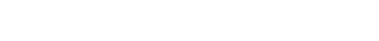And now the interesting part: this integral is the same we had at the beginning, so we can write an equation and solve for the integral: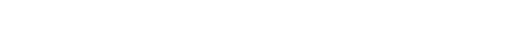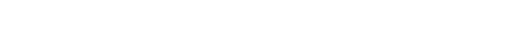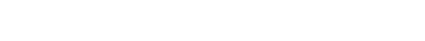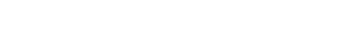Finally,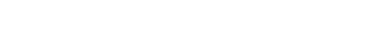Do you see anything familiar? If you know Euler’s identity,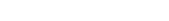you’ll notice that, if a = i,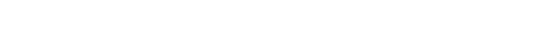This is in fact the condition in order for the integral to exist: a must not be equal to i. So if you find this integral (with a = i), you won’t have to solve it, because the answer is negative infinity.

Hope you liked this post, and if so, give it a like! If you have any questions leave a comment and I’ll be happy to help! The next post will be about the integral from 0 to infinity of the sinc function, i.e. sin(x)/x. Subscribe to stay updated!

Join 31 other subscribers.